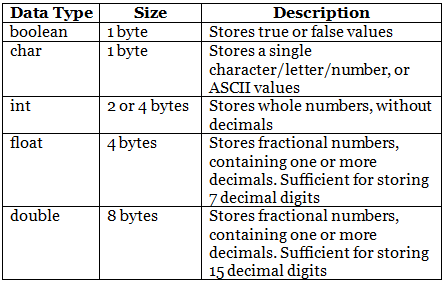C++ User Input & Data Types

# C++ User Input & Data Types - Notes | Study C++ Programming for Beginners - Class 8

 Table of contentsC++ Data Types1 Crore+ students have signed up on EduRev. Have you?

C++ User Input

• You have already learned that cout is used to output (print) values. Now we will use cin to get user input.
• cin is a predefined variable that reads data from the keyboard with the extraction operator (>>).
• In the following example, the user can input a number, which is stored in the variable x. Then we print the value of x:

Example

int x;

cout << "Type a number: "; // Type a number and press enter

cin >> x; // Get user input from the keyboard

cout << "Your number is: " << x; // Display the input value

Good To Know

• cout is pronounced "see-out". Used for output, and uses the insertion operator (<<)
• cin is pronounced "see-in". Used for input, and uses the extraction operator (>>)

Creating a Simple Calculator

In this example, the user must input two numbers. Then we print the sum by calculating (adding) the two numbers:

Example

int x, y;

int sum;

cout << "Type a number: ";

cin >> x;

cout << "Type another number: ";

cin >> y;

sum = x + y;

cout << "Sum is: " << sum;

There you go! You just built a basic calculator!

C++ Data Types

As explained in the Variables chapter, a variable in C++ must be a specified data type:

Example

int myNum = 5;               // Integer (whole number)

float myFloatNum = 5.99;     // Floating point number

double myDoubleNum = 9.98;   // Floating point number

char myLetter = 'D';         // Character

bool myBoolean = true;       // Boolean

string myText = "Hello";     // String

Basic Data Types

The data type specifies the size and type of information the variable will store:Numeric Types

Use int when you need to store a whole number without decimals, like 35 or 1000, and float or double when you need a floating point number (with decimals), like 9.99 or 3.14515.

int

int myNum = 1000;

cout << myNum;

float

float myNum = 5.75;

cout << myNum;

double

double myNum = 19.99;

cout << myNum;

float vs. double

The precision of a floating point value indicates how many digits the value can have after the decimal point. The precision of float is only six or seven decimal digits, while double variables have a precision of about 15 digits. Therefore it is safer to use double for most calculations.

Scientific Numbers

A floating point number can also be a scientific number with an "e" to indicate the power of 10:
Example

float f1 = 35e3;

double d1 = 12E4;

cout << f1;

cout << d1;

Boolean Types

A boolean data type is declared with the bool keyword and can only take the values true or false.
When the value is returned, true = 1 and false = 0.

Example

bool isCodingFun = true;

bool isFishTasty = false;

cout << isCodingFun;  // Outputs 1 (true)

cout << isFishTasty;  // Outputs 0 (false)

Character Types

The char data type is used to store a single character. The character must be surrounded by single quotes, like 'A' or 'c':

Example

Alternatively, you can use ASCII values to display certain characters:
Example

char a = 65, b = 66, c = 67;

cout << a;

cout << b;

cout << c;

String Types

The string type is used to store a sequence of characters (text). This is not a built-in type, but it behaves like one in its most basic usage. String values must be surrounded by double quotes:
Example

string greeting = "Hello";

cout << greeting;

To use strings, you must include an additional header file in the source code, the <string> library:

Example

// Include the string library

#include <string>

// Create a string variable

string greeting = "Hello";

// Output string value

cout << greeting;

The document C++ User Input & Data Types - Notes | Study C++ Programming for Beginners - Class 8 is a part of the Class 8 Course C++ Programming for Beginners.
All you need of Class 8 at this link: Class 8

## C++ Programming for Beginners

15 videos|20 docs|13 tests
 Use Code STAYHOME200 and get INR 200 additional OFF

## C++ Programming for Beginners

15 videos|20 docs|13 tests

Track your progress, build streaks, highlight & save important lessons and more!

,

,

,

,

,

,

,

,

,

,

,

,

,

,

,

,

,

,

,

,

,

;